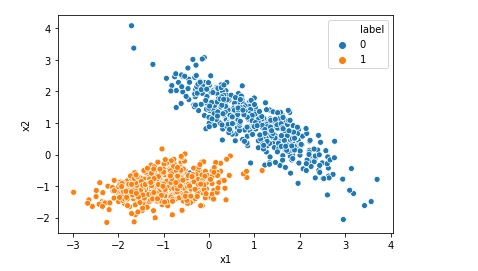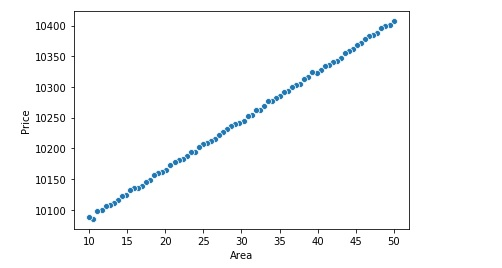# Difference Between Batch, Mini-Batch and Stochastic Gradient Descent

Gradient Descent is one the key algorithm used in Machine Learning. While training machine learning model, we require an algorithm to minimize the value of loss function. Gradient Descent is one of the optimization algorithm , that is used to minimize the loss. There are mainly three types of Gradient Descent algorithm1. Batch Gradient DescentBatch … Continue reading Difference Between Batch, Mini-Batch and Stochastic Gradient Descent

# How to write command-line arguments using argparse in python

If you want to run a .py python file from command line and you also want to pass the argument using command line you can use argparse library. This can be done as below: import argparse # Command Line arguments argp = argparse.ArgumentParser() argp.add_argument('--my_var', dest="my_var", action="store", type=int, default=5) params = argp.parse_args() myvar= params.my_var print(myvar) Then … Continue reading How to write command-line arguments using argparse in python# Handling missing data in pandas data frame python

In this post we are going to discuss how to handle missing data from a pandas data frame. Find total number of missing data in the data frame missing_total = df.isnull().sum().sum() Find number of missing data in each column in a data frame missing_per_column = df.isnull().sum() Investigate patterns in the amount of missing data in … Continue reading Handling missing data in pandas data frame python

# int object is not iterable Error

If your code is like below count = 100 for number in count: This will give you error like.. int object is not iterable. In Python, the thing you pass to a for statement needs to be some kind of iterable object. The variable count here is a number which is not iterable. You should … Continue reading int object is not iterable Error# Random Forest Classification in Python in 10 Lines

Random Forest algorithm is like an ensemble algorithm made of Decision Trees, which comprises more than one decision tree to create a model. It creates more than one tree like conditional control statements to create its model hence it is named as Random Forest. Random Forest machine learning algorithm can be used to solve both … Continue reading Random Forest Classification in Python in 10 Lines# Random Forest Regression in Python in 10 Lines

Random Forest algorithm is like Decision Tree, which comprises more than one decision tree to create a model. Random Forest algorithm is an ensemble method. It creates more than one tree like conditional control statements to create its model hence it is named as Random Forest. Random Forest machine learning algorithm can be used to … Continue reading Random Forest Regression in Python in 10 Lines# Implementing Logistic Regression in 10 lines in Python

Logistic Regression is one of the most popular Machine Learning algorithm used for the classification problems. It should be noted that though there is a regression word in the name of the algorithm Logistic Regression, it is used for classification problems. A use case of Logistic regression could be, based on the symptoms for a disease that a patient has Logistic … Continue reading Implementing Logistic Regression in 10 lines in Python

# How to Enable Intellisense or Autocomplete in Jupyter Notebook

No matter how good you are in programming with respect to a language like python you may not be able to remember all the functions names or syntax or function parameters. So you may require to use intellisense or autocomplete feature of Jupyter notebook while programming in pandas, python and similar libraries. In the below … Continue reading How to Enable Intellisense or Autocomplete in Jupyter Notebook

# How to Disable Warnings in Python and Pandas

Many a times when you run Python code in pandas you get warnings like below Disable or filter or suppress warning in python pandas However for various reasons you may want to disable or filter these warnings. For that use the below code import warnings warnings.filterwarnings("ignore") This will disable all the warnings and code will … Continue reading How to Disable Warnings in Python and Pandas# Linear Regression using sklearn in 10 lines

Linear regression is one of the most popular and fundamental machine learning algorithm. If relationship between two variables are linear we can use Linear regression to predict one variable given that other is known. For example if we are researching how the price of the house will vary if we change the area of the … Continue reading Linear Regression using sklearn in 10 lines